﻿ 炎黄子孙的“共旋”弦理论与土星光环的形成机制 Chinese Strings “Resonance” Theory and the Formation Mechanism of Saturn’s Rings

Astronomy and Astrophysics
Vol.05 No.04(2017), Article ID:22482,16 pages
10.12677/AAS.2017.54005

Chinese Strings “Resonance” Theory and the Formation Mechanism of Saturn’s Rings

Yueming Wu

Hangzhou Normal University, Hangzhou Zhejiang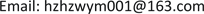Received: Oct. 9th, 2017; accepted: Oct. 18th, 2017; published: Oct. 27th, 2017ABSTRACT

“Resonance” string theory is a basic theory, which Albert Einstein once pursued, to explore the unity of gravitation and electromagnetic force by using physical method. In the process of deducing the formulas of “Resonance” string theory by means of the “simple string” method, it is found that nature endow all moving matter with the function of “self-replication”. It is believed that both of the gravitational waves and electromagnetic waves are resulted from the “self-replicating” functions of the planet’s rotation. It is also thought that Saturn and the crushed ice forming the light ring consist of electric dipole. The formation mechanism of Saturn’s rings can be explained by the “resonance” string theory. At the same time, it also suggests that the theory has been validated by nature.

Keywords:“Resonance” String Theory, Self-Replication, Electric Dipole, Light Ring, Mechanism“共旋”弦理论是用物理方法探索爱因斯坦曾经追寻的引力与电磁力相统一的基础理论。在运用“简弦量”'物理方法推导“共旋”弦理论公式过程中，发现大自然赋予所有运动物质具有“自我复制”功能。认为引力波和电磁波(光波)均是星球'自转运动'的“自我复制”功能所致，土星和组成光环的碎冰块均由电偶极子组成，“共旋”弦理论在解释土星光环的形成机制的同时，也说明“共旋”弦理论得到大自然的验证。Copyright © 2017 by author and Hans Publishers Inc.1. 引言

“卡西尼号”土星探测器，在1997年10月15日发射升空。经过6年8个月、35亿千米的漫长太空旅行之后，已于2004年7月1日按计划顺利进入环绕土星转动的轨道；2004年以来，“卡西尼”号探测器一直在围绕土星的轨道上运行，对其本体、卫星以及光环系统进行迄今为止最为细致的考察。2017年9月，“卡西尼”号探测器将结束使命，进行它的“自杀任务”——俯冲入土星内环，并将关键数据传回地球，随后在土星大气层分崩离析。此事令人产生一种“悲壮”之情。我们为美国宇航局科学家的“精密计算”、“精准调控”的高超技术而欢呼！也为“卡西尼号”的“即将逝世”而惋惜；深切体会到当前基础科学理论跟不上科学技术的飞速发展，至今人类还不能科学解释行星环的形成机理，我们必须遵循“自由畅想、大胆假设、认真求证。”的科学方法和“百花齐放、百家争鸣”的科学方针进行探索。

2. “共旋”理论对引力(惯性、质量)本质的探索

“物理学对于简谐量情有独钟，这缘于简谐量具有若干独特的性质。……对于弹性系统的强迫振动的处理方法，就是其应用的一个典型。”  自旋的星球(如太阳、地球等)运动之所以能亿年不停，就是这样一种非线性的有阻尼的自激振动弹性系统，若该自旋系统中有一质量 ${m}_{i}$ 的质点(见图1自旋系统的共旋示意图)，当其绕转轴自旋时会产生一个向轴(心)力。该力作为自旋系统的周期性强迫力作用于系统的质点自身，该力的角频率也为 ${\omega }_{0}$ ，即 $\omega ={\omega }_{0}$ ，驱动力(向心力)之幅值为： ${H}_{i}={m}_{i}{r}_{i}{\omega }_{0}^{2}\mathrm{sin}\left(\theta \right)$ ，相位呈周期性变化，因此该力呈周期性变化，该力可认为是以自转周期为周期的强迫力。系统在周期性外力作用下作受迫振动。该类系统可用“范·德·波耳(Van der pol)方程(简称为VDP方程)求解。VDP方程是由20世纪20年代由范·德·波耳在研究电子管振荡和模拟人的心脏搏动的基础上提出的。”  来表述：

$\frac{{\text{d}}^{2}x}{\text{d}{t}^{2}}-\mu \left({x}_{0}^{2}-{x}^{2}\right)\frac{\text{d}x}{\text{d}t}+{\omega }_{0}^{2}x+H\mathrm{cos}\omega t=0$ (1)

${m}_{i}\frac{{\text{d}}^{2}x}{\text{d}{t}^{2}}+\gamma \frac{\text{d}x}{\text{d}t}+{\omega }_{0}^{2}x+H\cdot \mathrm{cos}\omega t=0$ (2)

$2\beta =\frac{\gamma }{{m}_{i}}$$h=\frac{H}{{m}_{i}}$ ，整理(2)式得：

$\frac{{\text{d}}^{2}x}{\text{d}{t}^{2}}+2\beta \frac{\text{d}x}{\text{d}t}+{\omega }_{0}^{2}x+h\cdot \mathrm{cos}\omega t=0$ (3)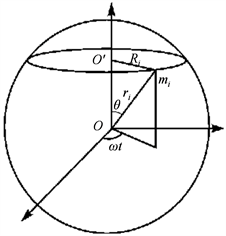Figure 1. Schematic diagram of spin system

$x={A}_{0}{\text{e}}^{-\beta \cdot t}\mathrm{cos}\left({\omega }_{0}t+\delta \right)+A\cdot \mathrm{cos}\left(\omega t+\phi \right)$ (4)

$x=A\cdot \mathrm{cos}\left(\omega t+\phi \right)$ (5)

$A=\frac{h}{\sqrt{{\left({\omega }_{0}^{2}-{p}^{2}\right)}^{2}+4{\beta }^{2}{p}^{2}}}$${\phi }_{0}=\mathrm{arctan}\frac{-2\beta \omega }{{\omega }_{0}^{2}-{\omega }^{2}}$ (6)

${\omega }_{r}=\sqrt{{\omega }_{0}^{2}-2{\beta }^{2}}$ , ${A}_{M}=\frac{h}{2\beta \sqrt{{\omega }_{0}^{2}-{\beta }^{2}}}$ (7)

${\omega }_{r}\approx {\omega }_{0}$ , ${A}_{M}\approx \frac{h}{2\beta {\omega }_{0}}$ (8)

$B\left(\omega \right)=\omega A\left(\omega \right)$ , ${{\phi }^{\prime }}_{0}\left(\omega \right)={\phi }_{0}\left(\omega \right)+\text{π}/2$ (9)

$\because {h}_{i}=\frac{{H}_{i}}{{m}_{i}}={r}_{i}{\omega }_{0}^{2}\mathrm{sin}\left(\theta \right)$${A}_{i}=\frac{{h}_{i}}{2\beta {\omega }_{0}}$ ，故有： ${A}_{i}=\frac{{m}_{i}{r}_{i}{\omega }_{0}\mathrm{sin}\left(\theta \right)}{2\beta }$ ；整个系统的总向轴(心)力振幅为：

$A=\underset{i=1}{\overset{n}{\sum }}{m}_{i}\cdot \frac{{r}_{i}{\omega }_{0}\mathrm{sin}\left[\theta \right]}{2\beta }$ ; (10)

$M=\rho \left(4\text{π}{R}^{3}/3\right)$ ; ∴ $A=\frac{\text{3π}R{\omega }_{0}M}{\text{32}\beta }$ ; (11)

${E}_{p}=A\cdot \mathrm{cos}\left[{\omega }_{0}\cdot \left(t-\frac{r}{c}\right)\right]/r$ . (12)

$\begin{array}{c}F=-\nabla {E}_{p}=\left(\frac{\partial }{\partial r}{e}_{r}+\frac{1}{r}\frac{\partial }{\partial \theta }{e}_{\theta }+\frac{1}{r\cdot \text{sin}\theta }\frac{\partial }{\partial \phi }{e}_{\phi }\right)\frac{A}{r}\text{cos}\left[{\omega }_{0}\left(t-\frac{r}{c}\right)\right]\\ =\frac{A\cdot \text{cos}\left[{\omega }_{0}\cdot \left(t-\frac{r}{c}\right)\right]}{{r}^{2}}-\frac{A\cdot {\omega }_{0}\cdot \text{sin}\left[{\omega }_{0}\left(t-\frac{r}{c}\right)\right]}{r\cdot c}\end{array}$ (13)

$F=\frac{A}{{r}^{2}}\left\{\text{cos}\left[{\omega }_{0}\left(t-\frac{r}{c}\right)\right]-\frac{r{\omega }_{0}}{c}\text{sin}\left[{\omega }_{0}\left(t-\frac{r}{c}\right)\right]\right\}$ (14)

${\omega }_{0}=\frac{2\text{π}}{27.28×24×3600}=2.66576×{10}^{-6}\text{rad}/\text{s}$ ；太阳引力波波长： ${\lambda }_{s}=7.07×{10}^{14}\text{m}$ ；故太阳系八大行星均在

$G=\frac{3\text{π}R{\omega }_{0}}{32\beta }\left(\mathrm{cos}\left[{\omega }_{0}\left(t-\frac{r}{c}\right)\right]-\frac{r{\omega }_{0}\mathrm{sin}\left[{\omega }_{0}\left(t-\frac{r}{c}\right)\right]}{c}\right)$ ;(15)

$\mathrm{cos}\left[{\omega }_{0}\left(t-\frac{r}{c}\right)\right]=\mathrm{cos}\left[{\omega }_{0}t\right]\mathrm{cos}\left[\frac{{\omega }_{0}r}{c}\right]+\mathrm{sin}\left[{\omega }_{0}t\right]\mathrm{sin}\left[\frac{{\omega }_{0}r}{c}\right]$ (16)

$\mathrm{sin}\left[{\omega }_{0}\left(t-\frac{r}{c}\right)\right]=\mathrm{cos}\left[{\omega }_{0}t\right]\mathrm{sin}\left[\frac{{\omega }_{0}r}{c}\right]-\mathrm{sin}\left[{\omega }_{0}t\right]\mathrm{cos}\left[\frac{{\omega }_{0}r}{c}\right]$ (17)

$\mathrm{cos}\left[{\omega }_{0}\left(t-\frac{r}{c}\right)\right]={\left(\mathrm{cos}\left[{\omega }_{0}t\right]\right)}^{2}+{\left(\mathrm{sin}\left[{\omega }_{0}t\right]\right)}^{2}=1$ (18)

$\mathrm{sin}\left[{\omega }_{0}\left(t-\frac{r}{c}\right)\right]=\mathrm{cos}\left[{\omega }_{0}t\right]\mathrm{sin}\left[{\omega }_{0}t\right]-\mathrm{sin}\left[{\omega }_{0}t\right]\mathrm{cos}\left[{\omega }_{0}t\right]=0$ (19)

$F=\frac{GM}{{r}^{2}}$ (20)

(20)式就是牛顿的万有引力公式，是物体的相互作用力的普适的公式。它概括了人世间显现的物体间的相互作用，但它忽略了时间过程，给人以超距作用的感觉。

3. 运用物理方法探索爱因斯坦追求的统一理论

${F}_{yl}=\frac{A}{{r}^{2}}\mathrm{cos}\left[{\omega }_{0}\left(t-\frac{r}{c}\right)\right]$ (21)

${F}_{dl}=\frac{A}{{r}^{2}}\left(\frac{-R{\omega }_{0}}{c}\mathrm{sin}\left[{\omega }_{0}\left(t-\frac{r}{c}\right)\right]\right)$ (22)

${F}_{dl}=\frac{3\text{π}{\omega }_{0}RM}{32\beta {r}^{2}}\left({\left(\frac{R{\omega }_{0}}{c}\right)}^{2}\mathrm{cos}\left[{\omega }_{0}t\right]\right)$ (23)

2008年，中国基础科学杂志的探索争鸣专栏刊载陈永明的《质电类比当量和引力波》的文章。陈永明认为：“库仑定律和万有引力定律具有完全相同的形式，因而我们可以借用库仑定律来计算两质点间万

$\lambda =\sqrt{\frac{G}{K}}=±8.6127×{10}^{-11}\left(\text{C}/\text{kg}\right)$ (24)” 

“共旋”理论认为引力不会产生磁场，只有运动的电荷才能产生磁场。认为不同物质结构星球有不同的内秉系数。非导电体物质结构星球的自转运动会产生引力波，导电体物质结构星球的自转运动不仅会产生引力波还会形成电偶极子星球产生电磁波。

$G$$K$ 的公式相似，不同的是 $\beta$ 数值的改变。因此(23)式的电偶极子星球产生正负电荷产生电力的公式为：

${F}_{dl}=\frac{{\lambda }^{2}Mm}{{r}^{2}}{\left(\frac{{\omega }_{0}R}{c}\right)}^{2}\mathrm{cos}\left[{\omega }_{0}t\right]$ (25)

${F}_{dl}=\frac{{Q}_{z+}{Q}_{z-}}{{r}^{2}}\mathrm{cos}\left[{\omega }_{0}t\right]$ (26)

$\varphi =\frac{p\cdot r}{4\text{π}{\epsilon }_{0}{r}^{3}}$ (27)

$\varphi =\frac{p\cdot e}{4\text{π}{\epsilon }_{0}{r}^{2}}=\frac{p}{4\text{π}{\epsilon }_{0}{r}^{2}}\mathrm{cos}\left[\theta \right]$ (28)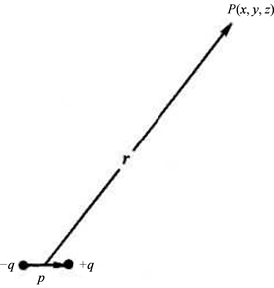Figure 2. Electric dipole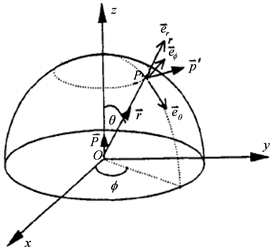Figure 3. Electrical dipole radiation schematic diagram

$\begin{array}{c}E=-\nabla \varphi =-\left(\frac{\partial }{\partial r}{e}_{r}+\frac{1}{r}\frac{\partial }{\partial \theta }{e}_{\theta }+\frac{1}{r\mathrm{sin}\left[\theta \right]}\frac{\partial }{\partial \phi }{e}_{\phi }\right)\frac{p}{4\text{π}{\epsilon }_{0}{r}^{2}}\mathrm{cos}\left[\theta \right]\\ =\frac{p}{4\text{π}{\epsilon }_{0}{r}^{2}}\left(2\mathrm{cos}\left[\theta \right]{e}_{r}+\mathrm{sin}\left[\theta \right]{e}_{\theta }\right)\end{array}$ (29)

(28)式中， $\theta$ 是场点 $p\left(r\right)$ 的位矢 $r$$z$ 轴的夹角， $\phi$ 是位矢 $r$ 与xoy平面的投影与x轴的夹角， ${e}_{r}$${e}_{\theta }$${e}_{\phi }$$p\left(r\right)$ 点的单位矢量。” 

(26)式为“共旋起电”产生“电偶极子”电荷在空间激发的电场对单位电荷的电力公式，则有：

${E}_{\phi }=\frac{{F}_{dl}}{{q}_{0}}=\frac{{Q}_{z+}{Q}_{z-}}{{q}_{0}{r}^{2}}\mathrm{cos}\left[{\omega }_{0}t\right]$ ；取式中 $\mathrm{cos}\left[{\omega }_{0}t\right]$${\omega }_{0}$ 矢量的方向为电偶极子的方向，则由 ${Q}_{z+}{Q}_{z-}$ 组成的，正负电荷间距 $2\cdot {R}_{0}$ 的电偶极子星球电矩为： $p={\lambda }^{2}Mm{\left(\frac{{\omega }_{0}{R}_{0}}{c}\right)}^{2}={\lambda }^{2}\frac{Mm{\omega }_{0}^{2}{R}_{0}2{R}_{0}}{2{c}^{2}}$ ；则在球面坐标中，电偶极子在空间任一点 $p\left(r\right)$ 激发电场的公式为：

${E}_{\phi }=\frac{p\cdot r}{2{q}_{0}{r}^{3}}$ (30)

$p={\lambda }^{2}Mm{\left(\frac{{\omega }_{0}{R}_{0}}{c}\right)}^{2}$${\epsilon }_{0}=\frac{{q}_{0}}{2\text{π}}$ (31)

(31)式的物理意义是：因为 $p\propto Mm{\omega }_{0}^{2}{R}_{0}$ ，可见自转星球电偶极子是自转星球每个质点向心力自我复制后的叠加所形成。真空介电常量 ${\epsilon }_{0}$ (或真空电容率)可理解为单位体积里所容纳的单位电量。可见由导电物质构成的自旋星球会“共旋”起电，形成电偶极子星球。

2012年以后，根据培根倡导的科学理论必须符合“物理模型、数学支撑。实验验证”三标准，笔者认为太阳系的自旋星球均为电偶极子星球。以地球为例，地球的铁镍金属内核会“共旋”起电，在地核面的不同卦面产生不同电荷、形成电偶极子，由于正负电荷的不同电位；产生涡电流使外核熔融成为液态。由于地幔是由氧、硅、镁等物质构成，是一个大电容，使地幔近液核的界面带正电荷，幔壳面带负电荷；电荷的转动，形成了地球磁场。这是地球热能之源、电能之源、也是磁能之源。由于地核不同卦面电位不同，在产生涡电流的同时，也向空间泄漏，使地球保持一个带负电的准静电球体。“地球的晴天大气电场的变化，经测定晴天电场的变化与地方时无关，即全球大气电场的变化是同步地发生，一天之内，大约在格林威治时间18:00左右出现极大值，在4:00左右出现极小值。”  其原因就来源于地球的地核电偶极子通过西藏高原珠峰的“尖端放电”所形成。

4. 中国人提出了“道法自然”弦理论公式

2007年笔者参加了中国地球物理学会天灾预测专业委员会，在天灾预测实践过程中，认识到地球人应加强对地球电磁场的研究。2012年笔者运用物理方法统一了引力场和电磁场，得到“脉冲星”天然实验室的检验。并提出了“共旋”弦理论。从哲学高度分析“共旋”弦理论公式(14)，表明世界是物质的、物质是运动的，运动具有“自我复制”功能，其规律是可以运用数学式描述。共旋理论称式(14)为宇宙公式(左旋、右旋宇宙都适用)。

$F=W\left\{\mathrm{cos}\left[\psi \right]-Y\mathrm{sin}\left[\psi \right]\right\}$ ; (32)

$F=\frac{GMm}{{r}^{2}}\mathrm{cos}\left[\psi \right]±\frac{KMm}{{r}^{2}}Y\mathrm{sin}\left[\psi \right]$ ; (33)

(33)式是炎黄子孙提出的“道法自然”弦理论公式。是一个描述引力及电力本质及其产生机制(包括质量、惯性)的宇宙公式，也是可描述四种基本力(引力、弱力、电磁力和强力)相互作用规律的统一理论公式。公式非常简洁和壮丽。杨振宁在他的《美与物理学》中谈到对狄拉克的文章感受时说：“数学的最高境界是结构美，是简洁的逻辑美，因此他的文章也就是给读者‘秋水文章不染尘’”的感受。杨先生在对牛顿、麦克斯韦、爱因斯坦、狄拉克、海森堡等人的方程式评价时说：“它们以极度浓缩的数学语言写出了物理世界的基本结构，可以说是造物者的诗篇。” 

5. 土星光环的成因探索

2005年笔者在《共旋理论初探(下)》一书中，对“土星光环动力学机制探索”一节中是这样写的：“从地球上看土星环，似乎是个静止不动的整体结构，但在1859年麦克斯韦就已经从理论上证明整个的固体或液体环都不能稳定地围绕着土星旋转，它必定是由许多小碎块组成，后来的宇航探测证实这一点，发现了土星的环中有缝、缝中有环的复杂结构，并且离土星近的物质运动得慢，远的反倒运动得快。这显然与天体在引力支配下的作开普勒转动不符。因此共旋理论认为除引力外，还应考虑土星的共旋梯力和土星电磁场的洛伦兹力的共同作用。”认为：“这些物质(碎块、冰块)在土星赤道面上，其受指向赤道面的共旋梯力为零；在磁赤道面上受磁场方向的洛伦兹力也为零，因土星自转轴与磁轴基本重合，故这些固体物质在共同赤道面上最为稳定，它们会因有不同质量、不同物质成分和带有不同电量，而分布在不同轨道上。在共旋梯力和洛伦兹力的共同作用下，它们用比土星自旋更快的速度环绕土星竟相奔驰，形成大自然的艺术珍品—美丽的光环。”  书中只有定性描述、没有定量计算。

5.1. 光环轨道运动角速度(转速)比土星自旋更快的物理机制探讨

${T}_{行}^{2}\left({m}_{太}+{m}_{行}\right)G=4{\text{π}}^{2}{a}_{行}^{3}$ (34)

${T}_{卫}^{2}\left({m}_{行}+{m}_{卫}\right)G=4{\text{π}}^{2}{a}_{卫}^{3}$ (35)

$\frac{{m}_{太}+{m}_{行}}{{m}_{行}+{m}_{卫}}=\left(\frac{{a}_{行}^{3}}{{a}_{卫}^{3}}\right)\cdot \left(\frac{{T}_{卫}^{2}}{{T}_{行}^{2}}\right)$ (36)

$\frac{{m}_{太}}{{m}_{行}}=\left(\frac{{a}_{行}^{3}}{{a}_{卫}^{3}}\right)\cdot \left(\frac{{T}_{卫}^{2}}{{T}_{行}^{2}}\right)$ ; (37)

${\omega }_{卫}=\frac{2\text{π}}{{T}_{卫}}=\frac{1.9473×{10}^{8}}{\sqrt{{a}_{卫}^{3}}}$ ;

Plot[ ${\omega }_{卫}$ ,{ ${a}_{卫}$ , $1×{10}^{7}$ , $1×{10}^{10}$ }]：

5.2. 光环电偶极子的成因系离心力所为

$\lambda$ 和光速的等有关数据代入电偶极子的带电量公式： ${Q}_{tx}=±\lambda {M}_{tx}\frac{{\omega }_{tx}{R}_{tx}}{c}$ ；式中：

$\lambda =±8.6127×{10}^{-11}\left(\text{C}/\text{kg}\right)$ ，质量： ${M}_{tx}=5.685×{10}^{26}\text{kg}$ ；半径： ${R}_{tx}=6.0268×{10}^{7}\text{m}$ ；自转速度：

${\omega }_{tx}=\text{2π}/\left(0.42639×24×3600\right)\left(\text{rad}/\text{s}\right)$ ；光速： $c=3×{10}^{8}\left(\text{m}/\text{s}\right)$ ；代入公式，计算得土星电偶极子的电量为： ${Q}_{tx}=±1.67798×{10}^{12}\left(\text{C}\right)$ ；电偶极矩有： ${p}_{tx}={Q}_{tx}2{R}_{tx}=2.02257×{10}^{20}\left(\text{C}\cdot \text{m}\right)$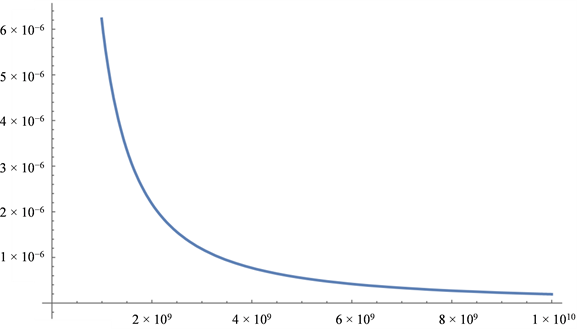Figure 4. The change of the ring speed of Kepler’s third law and the distance from Saturn

${P}_{1}={P}_{tx}$ ，土星电偶极子 ${P}_{1}$ 与光环电偶极子 ${P}_{2}$ (冰块电偶极子)的电偶极矩方向成相垂直的方向。

$ri{b}_{1}$$ri{b}_{2}$$rib$ 为B环中离土星距离最近、最远、以及平均半径距离的光环： $ri{b}_{1}=0.92×{10}^{8}\text{m}$$ri{b}_{2}=1.\text{175}×{10}^{8}\text{m}$ ；则： $rib=\left(ri{b}_{1}+ri{b}_{2}\right)/2=1.0475×{10}^{8}\text{m}$

$Tib=3.22656×{10}^{÷8}\sqrt{ri{b}^{3}}=34591.6\left(\text{s}\right)$ ;

$wib=2pi/Tib=0.000181639\left(\text{rad}/\text{s}\right)$ ;

${V}_{b}=2pi×rib×2.55×{10}^{7}×100=1.48×{10}^{18}\left({\text{m}}^{3}\right)$ ;

${p}_{b}={\lambda }^{2}\cdot {m}_{i}\cdot {\left(\frac{{\omega }_{i}{R}_{i}}{c}\right)}^{2}\cdot {V}_{b}=1.2378×{10}^{9}\left(\text{C}\cdot \text{m}\right)$ ;

$L=\frac{{P}_{tx}\cdot {P}_{b}}{4\text{π}\cdot {\epsilon }_{0}\cdot {r}^{3}}=1.9584×{10}^{15}\left(\text{N}\cdot \text{m}\right)$

5.3. 土星光环发光机制也是“向心力”的“自我复制”所为

6. 关注和讨论

1) 发现大自然赋予所有运动物质具有“自我复制”功能，运用简弦量物理方法推导得出的由星球自转运动产生力的(14)式，该力为共旋(振)力，可称为膺力(复制力)，也称之“惯性力”。因每个质点的自我复制只有一次，而整个自旋系统的质量是系统各质点质量之和，故可将系统质量称之为“惯性质量”，这也是“惯性本质”之所在。可以认为：该发现有助于认识当年牛顿称之为上帝所施的“第一推动力”。

2) 炎黄子孙的“共旋”弦理论公式： $F=W\left\{\mathrm{cos}\left[\psi \right]-Y\mathrm{sin}\left[\psi \right]\right\}$ ；是一个描述引力及电力本质及其产生机制的宇宙公式。它由四个字母组成，认为世界上只有一种力，称之为： $F$ ，它由： $W=\frac{3\text{π}R{\omega }_{0}M}{32\beta {r}^{2}}$$Y=\frac{R{\omega }_{0}}{c}$$\psi ={\omega }_{0}\left(t-\frac{r}{c}\right)$ 组成，公式非常简洁和壮丽。从哲学高度分析公式表明世界是物质的、物质是

3) “共旋”弦理论是用物理方法探索爱因斯坦晚年追寻引力与电磁力相统一的基础理论。它提出了

$F=\frac{GMm}{{r}^{2}}\mathrm{cos}\left[\psi \right]±\frac{KMm}{{r}^{2}}Y\mathrm{sin}\left[\psi \right]$ ；即统一引力和电力的统一理论公式。这是由炎黄子孙根据东方的太

4) “共旋”弦理论能对“光波”形成机制进行解释。认为引力波和电磁波(光波)均是星球、实体粒子旋转运动的“自我复制”功能所致。认为土星和组成光环的碎冰块均为电偶极子，“共旋”弦理论在解释土星光环的形成机制的同时，说明共旋弦理论得到大自然实验室的验证。

Chinese Strings “Resonance” Theory and the Formation Mechanism of Saturn’s Rings[J]. 天文与天体物理, 2017, 05(04): 29-44. http://dx.doi.org/10.12677/AAS.2017.54005

1. 1. 中共中央宣传部. 习近平总书记系列重要讲话读本[M]. 北京: 学习出版社, 人民出版社, 2014: 100, 104-105.

2. 2. 伍岳明. 地震是太阳系星球相互作用之结果[N]. 科技日报, “四川汶川大地震机理探索”专辑, 2008-06-05.

3. 3. 伍岳明, 曹明富. 共旋理论初探. 上册——共旋引力波理论探索. 下册——共旋起电能源理论探索[M]. 北京: 科学技术文献出版社, 2005: 1-6, 28-35, 44-37, 58-68, 79-80.

4. 4. 伍岳明, 曹明富. 引力波的三种作用方式——日全食期间的引力波实验[C]//中国地球物理学会第26届年会论文集, 2010: 709-710.

5. 5. 钟锡华, 周岳明. 力学[M]. 北京: 北京大学出版社, 2000: 51, 174-193.

6. 6. 彭芳麟, 管靖, 胡静, 卢圣治. 理论力学计算机模拟[M]. 北京: 清华大学出版社, 2002: 192-197.

7. 7. 郭汉英选集编委会. 郭汉英杂文选集[M]. 桂林: 广西师范大学出版社, 2013: 9-17.

8. 8. 周凤林, 王晓芜, 胡炳元. 改变昨天的世界[M]. 上海: 上海科技教育出版社, 2012: 51-54.

9. 9. 陈永明. 质电类比当量和引力波. 中国基础科学•探索争鸣, 2008, 10(1): 34-37.

10. 10. 倪忠楚. 两电偶极子间的相互作用能和相互作用力[J]. 高师理科学刊, 2003, 23(4): 30-34.

11. 11. 张三慧. 电磁学(第二版) [M]. 北京: 清华大学出版社, 1999: 117-118.

12. 12. 倪简白, 主编. 物理新论[M]. 长春: 长春出版社, 2013: 1-15.

13. 13. 胡中为, 萧耐园. 天文学教程[M]. 北京: 高等教育出版社, 2003: 251-252.

14. 14. 傅振堂. 电偶极子相互作用问题[J]. 空军电讯工程学院学报, 1997(1): 55-58.